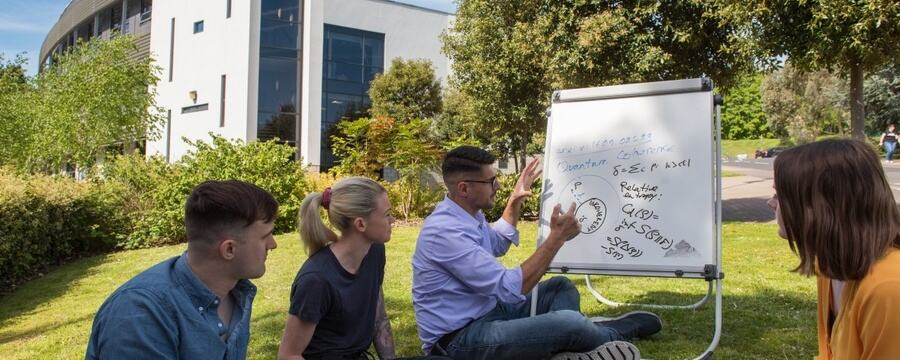New offer! Get 30% off your first 2 months of Unlimited Monthly. Start your subscription for just £35.99 £24.99. New subscribers only T&Cs apply

•# Problem solving solutions

In this article, the solutions to the questions posed in the previous step are shared.The solutions for the questions in the previous step are below. Use these to check your method and your answers.

## Question 1

A ball is thrown straight up, from 2 m above the ground, with a velocity of 15 m/s such that the height, (h) in metres at a given time (t) seconds can be represented as

[h= 2 +15t -5t^{2}]

a) By drawing a suitable graph estimate when the height is equal to 10 metres.

We first create a table of values that shows the values of (h) for various values of (t).
For example when (t=0), (h=2+15times 0 – 5 times 0^{2} = 2 m).
t 0 0.5 1 1.5 2 2.5 3 3.5
h 2 8.25 12 13.25 12 8.25 2 -6.75
We then plot these values of (h) and (t) on a graph as follows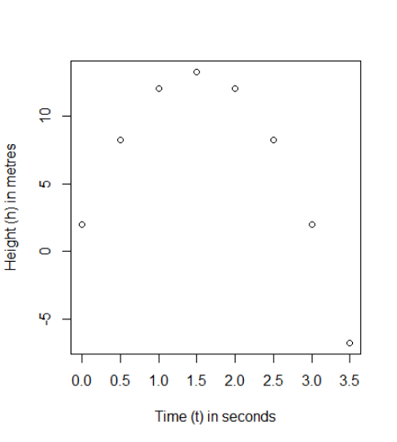We then draw a smooth curve through these points as follows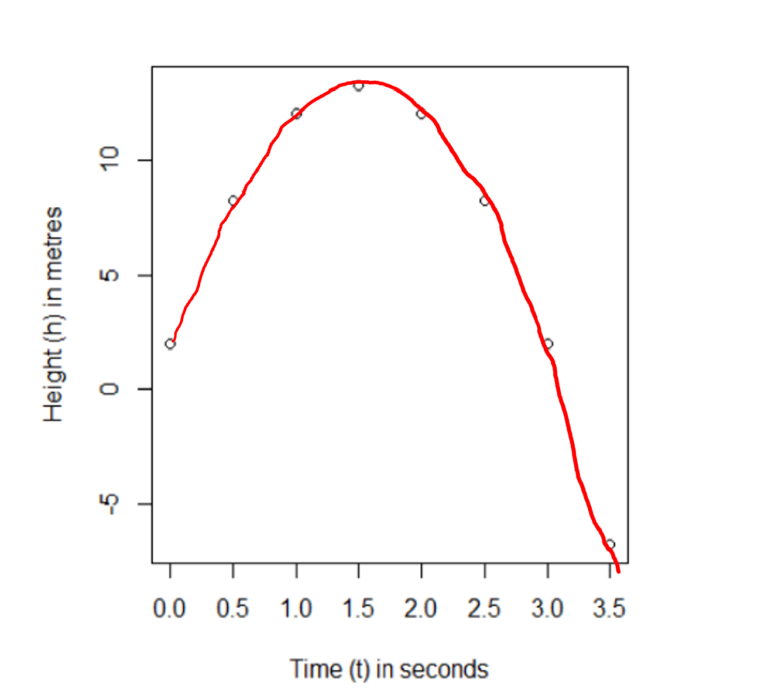We can then use the graph to find the values of (t) when (h=10) as follows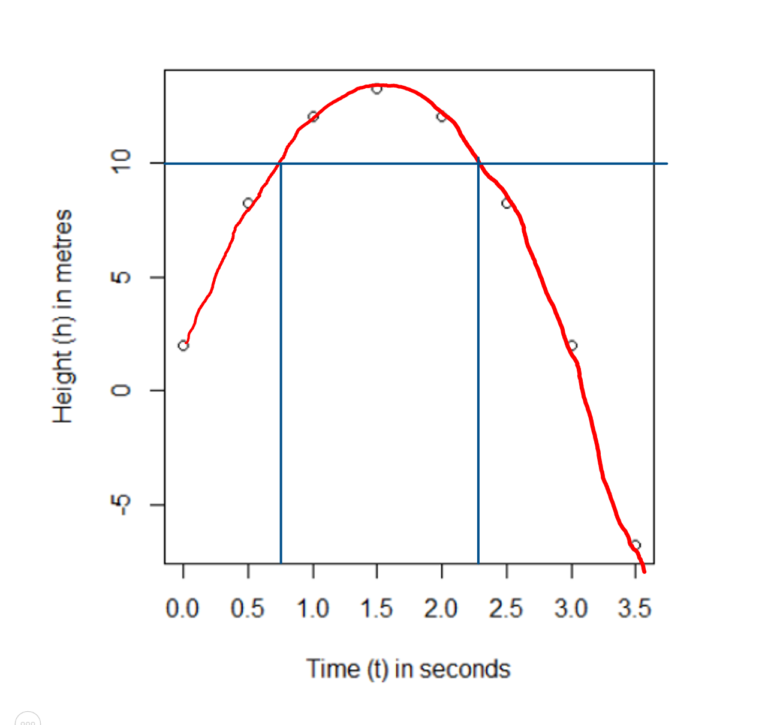Approximately when (h=10) (m), (t=0.75) (s) and (t=2.3) (s)
b) Algebraically, find when the ball hits the ground.
The question asks us to solve this question algebraically. Therefore we first write an equation for when the ball hits the ground. This is when (h=0) and so our equation is

[2+15t-5t^{2}=0]

To solve for (t) we use the quadratic equation which tells us that

[t=frac{-bpm sqrt{b^{2}-4ac}}{2a}]

In our equation (a=-5), (b=15) and (c=2), so that

[begin{eqnarray*} t=frac{-15pm sqrt{15^{2}-4times-5times 2}}{2times-5} =frac{-15pm sqrt{265}}{-10} = 3.13 text{ or } -0.128 end{eqnarray*}]

Therefore our solution is that the ball hits the ground at 3.13 seconds.
c) Find the maximum height and the time that the ball reaches this maximum height.
We use differentiation to find the maximum height by solving
(frac{dh}{dt}=0).
We find that (frac{dh}{dt}=15-10t) and therefore we need to solve

[begin{eqnarray*} 15-10t=0 15=10t t=1.5 end{eqnarray*}]

Then a stationary point is when (t=1.5) (s).
When (t=1.5), the height is
(h=2+15times 1.5 – 5times 1.5^{2}= 13.25) (m)
To confirm that this is a maximum height, we find the second derivative of (h) which is

[frac{d^{2}h}{dt^{2}}=-10]

This is less than 0 and therefore ((t,h)=(1.5,13.25)) is a maximum.

## Question 2

A scientist carries out three experiments to find the relationship between (x) and (y) in three different settings.
From experiment 1 you are given the following values of (x) and (y).
x 2 4 6
y 40 320 1080
The relationship between (x) and (y) is given by (y=Ax^{k}) for constants (A) and (k).
a) Use the laws of logarithm to rearrange (y=Ax^{k}) into the form (y’=mx’+c) where (y’=ln(y)) and (x’=ln(x)), and then using the values above, draw a suitable graph to find the values of (A) and (k).
We use the laws of logarithm to show that

[begin{eqnarray*} y=Ax^{k} ln(y)=ln(Ax^{k}) ln(y)=ln(A)+ln(x^{k}) ln(y)=ln(A)+kln(x) end{eqnarray*}]

such that (y’=mx’+c) where (y’=ln(y)), (x’=ln(x)), (m=k) and (c=ln(A)).
Then we find the values of (ln(y)) and (ln(x)) as in the following table
x 2 4 6
ln(x) 0.69 1.39 1.79
y 40 320 1080
ln(y) 3.69 5.77 6.98
and we plot these values on a graph as follows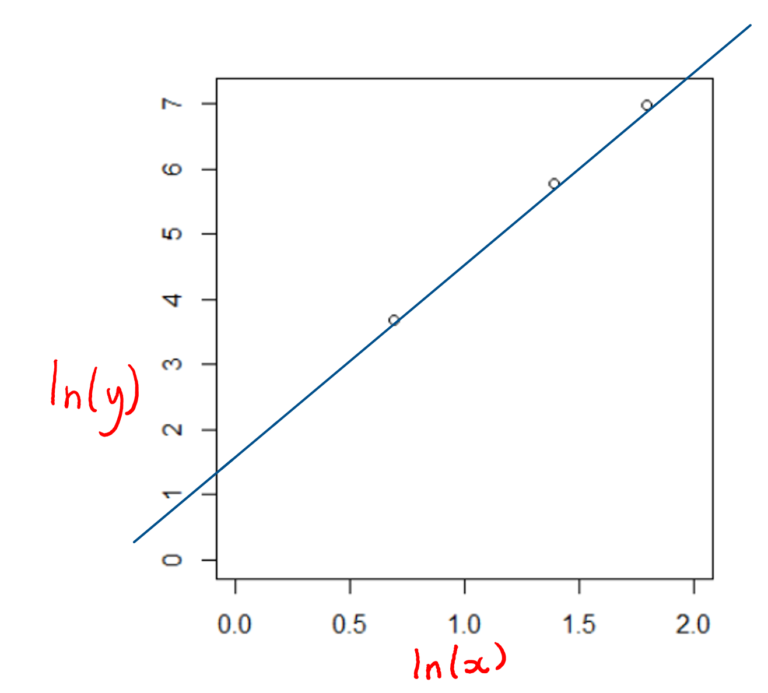We know that the relationship between (ln(y)) and (ln(x)) is (ln(y)=kln(x)+ln(A)), such that (k) is the gradient of the line and (ln(A)) is the y-intercept on the graph
We first find the gradient as

[k=m=frac{ln(y_{2})-ln(y_{1})}{ln(x_{2})-ln(x_{1})}=frac{6.98-3.69}{1.79-0.69}=3.0]

Using the graph we then find that the y-intercept is approximately 1.4 such that
(c=ln(A)=1.4).
Then we can find that
(A=e^{1.4}=4.1).
Therefore we have approximated that the relationship between (x) and (y) is (y=4.1x^{3})
For comparison the actual relationship is (y=5x^{3}).
From the second experiment the scientist informs you that when (x=4), (y=1280). From experiment 2 when (ln(x)) is plotted against (ln(y)) the resulting line is parallel is to the line using the data from experiment 1.
b) Find the relationship that connects (x) and (y) for experiment 2.
From part (a) we know that the relationship that connects (x) and (y) will be of the form (ln(y)=kln(x)+ln(A)).
The question tells us that the lines in experiment 1 and experiment 2 are parallel and therefore the gradients are equal. Therefore the value of (k) is 3. then the relationship for experiment 2 is

[ln(y)=3ln(x)+ln(A)]

By substituting the values of (x) and (y) that are given in the question into the formula we can find the value of (A)

[begin{eqnarray*} ln(1280)=3ln(4)+ln(A) ln(A)=ln(1280)-3ln(4) ln(A)=2.9957 A=e^{2.9957…} A=20 end{eqnarray*}]

Thus the relationship between (x) and (y) which has the form (y=Ax^{k}) can be found as (y=20x^{3}).
The scientist conducts experiment 3 and finds that when (x=1), (y=1). When the scientist plots (ln(x)) against (ln(y)) the resulting line is perpendicular to the lines from the data in experiment 1 and experiment 2.
c) Find the relationship that connects (x) and (y) for experiment 3.
The relationship that connects (x) and (y) is of the form (ln(y)=kln(x)+ln(A)).
The gradients of perpendicular lines, which we denote (m_{1}) and (m_{2}) are such that (m_{1}times m_{2}=-1). The gradient of the first line is 3 and therefore

[begin{eqnarray*} m_{1}times m_{2}=-1 3times m_{2}=-1 m_{2}=frac{-1}{3} end{eqnarray*}]

Therefore the value of (k) for experiment 3 is (frac{-1}{3}).
The relationship between (x) and (y) is of the form (y=Ax^{k}) and we know that (k=frac{-1}{3}), (x=1) and (y=1) so that

[begin{eqnarray*} 1=Atimes 1^{-1/3} 1=Atimes 1 A=1 end{eqnarray*}]

The relationship between (x) and (y) is (y=x^{-1/3})

## Question 3

a) A 5G mast is made up of two sections A and B as in the diagram below. Safety regulations mean that section A can be a maximum height of 50 m and section B can have a maximum height of 10m.
An inspector stands 25m from the mast and finds that the angle of elevation between the ground and the top of section A is (61^{o}) whilst the angle of elevation between the ground and the top of section B is (67^{o}). Does the mast meet the regulations?
Let’s first annotate the diagram with the angles and label the opposite and adjacent in the right angled triangles.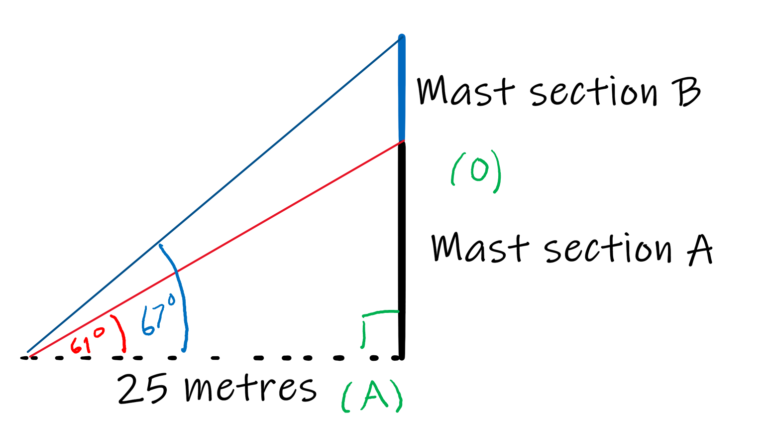The adjacent is equal to 25 metres in both triangles and we know that Opposite = (tan(theta) times) Adjacent. Then in the smaller triangle

[text{Opposite}=tan(61)times 25=45.1hspace{0.1cm}m]

Similarly for the larger triangle

[text{Opposite}=tan(67)times 25=58.9hspace{0.1cm}m]

Thus mast section A is 45.1 m and mast section B can be found as (58.9-45.1=13.8) m, as in the following diagram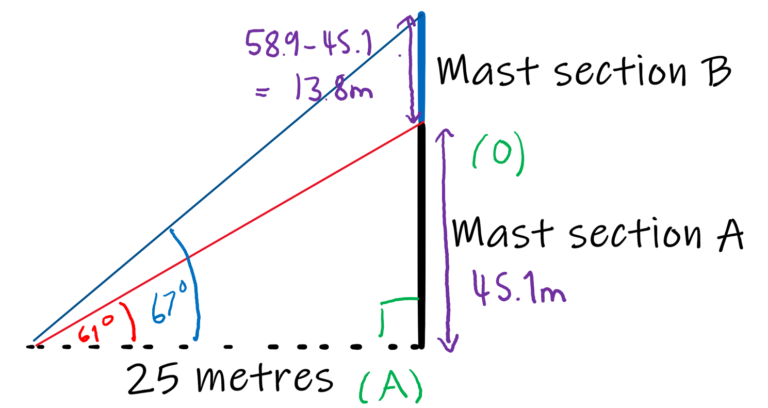The mast does not meet the regulations for section B as it exceeds the maximum height of 10 m.
b) Consider the amine (R-NH_{2}) below. The angle HNH is (109.3^{o}); the NH bond length is 103.8 pm. What is the interatomic separation between the H atoms?
Using the information in the question we have a triangle as follows and we need to find the length of side (a).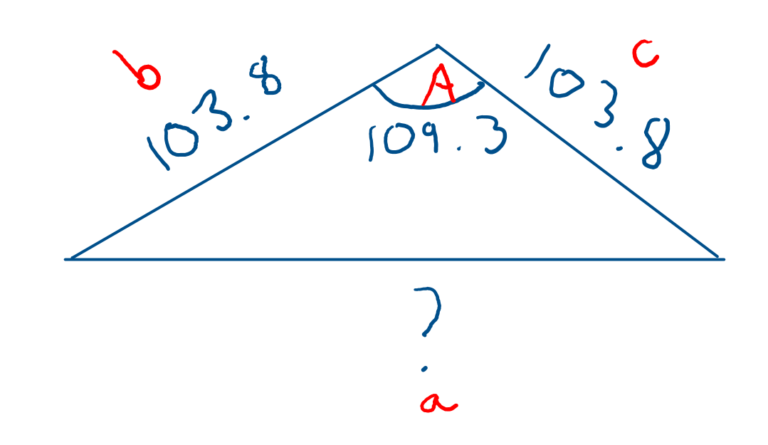We can use the cosine rule so that

[begin{eqnarray*} a^{2}=b^{2}+c^{2}-2bccos(A) a^{2}=103.8^{2}+103.8^{2}-2times 103.8 times 103.8 times cos(109.3) a^{2}=28671.09499 a=sqrt{28671.09499} a=169.3hspace{0.1cm}text{pm} end{eqnarray*}]

## Question 4

The displacement of a mass by a spring, in cm, after (t) seconds, can be modelled by the function (y(t)=4sin(3pi t -1)).
By drawing a suitable graph estimate the maximum displacement (amplitude), the time it takes for one complete cycle (period) and the cycles per second (frequency).
We first make a table of suitable values for (t) and (y). As an example when (t=0), (y(0)=4sin(3pitimes 0 -1)=-3.4)
t y
0 -3.4
0.1 -0.2
0.2 3.1
0.3 3.9
0.4 1.5
0.5 -2.2
0.6 -4.0
0.7 -2.5
0.8 1.0
0.9 3.7
1.0 3.4
We then plot the values of (t) and (y) and join the points with a smooth curve as in the graph below.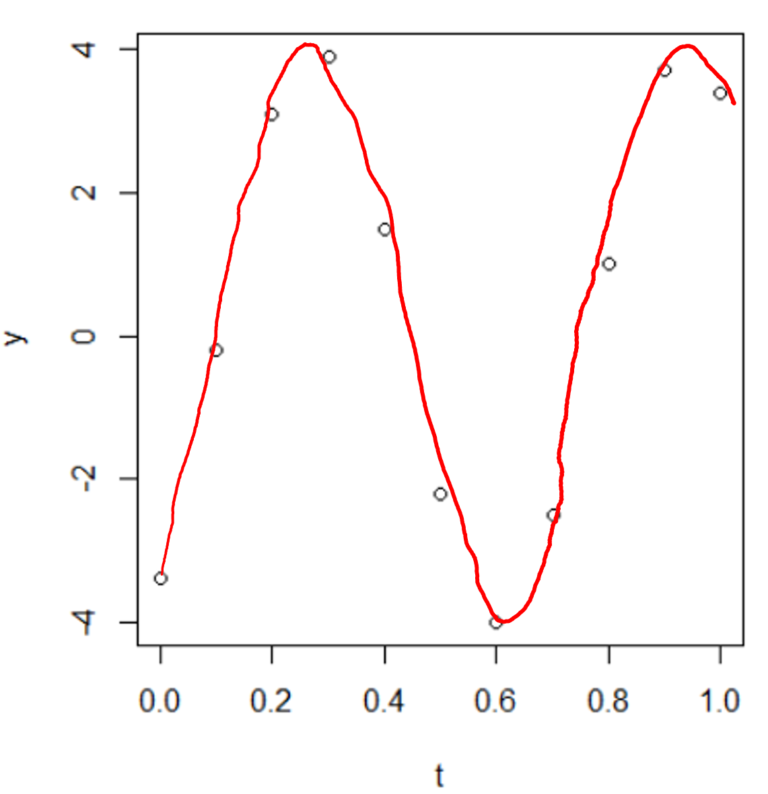By annotating the graph we can estimate the amplitude and period.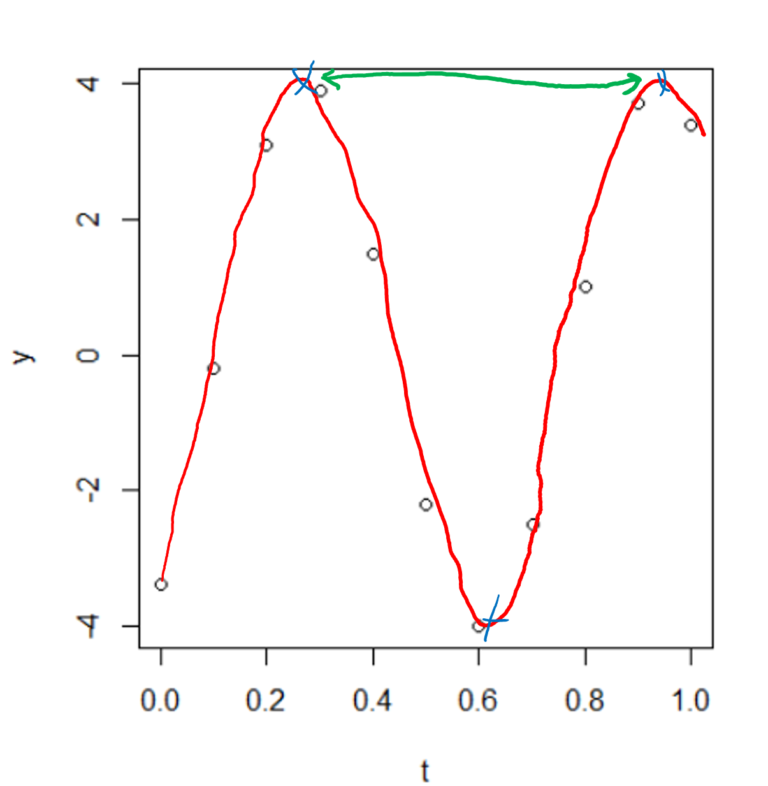The amplitude is approximately 4 cm.
The period is approximately 0.95-0.28=0.67.
The frequency is (1divtext{ period}) and (1div 0.67=1.5).

## Question 5

The number of births of a species of insect between (t_{1}) and (t_{2}) days can be found by finding the area bounded by the lines (y=0), (x=t_{1}), (x=t_{2}) and (y=10e^{0.04x}).
a) Find the number of births between day 20 and day 22.
When (20leq x leq 22) we see that (y>0) and thus

[begin{eqnarray*} int_{20}^{22} 10e^{0.04x}dx = left[frac{10e^{0.04x}}{0.04}right]_{20}^{22} =left(frac{10e^{0.04times 22}}{0.04}right)-left(frac{10e^{0.04times 20}}{0.04}right) =46.34 end{eqnarray*}]

Similarly the number of deaths of a species of insects between (t_{1}) and (t_{2}) days can be found by finding the area bounded by the lines (y=0), (x=t_{1}), (x=t_{2}) and (y=0.1xe^{0.2x}).
b) What is the overall population growth between day 5 and day 15?
For the number of births between day 5 and day 15 we calculate

[begin{eqnarray*} int_{5}^{15}10e^{0.04x}dx=left[frac{10e^{0.04x}}{0.04}right]_{15}^{5} =frac{10}{0.04}left(e^{0.04times 15}-e^{0.04times 5}right) =150.18 end{eqnarray*}]

Similarly for the number of deaths we need to calculate (int_{5}^{15}0.1xe^{0.2x}dx)
We use integration by parts. The formula for integration by parts is

[inthspace{0.1cm}uhspace{0.1cm}frac{dv}{dx}hspace{0.1cm}dx = uv – int vhspace{0.1cm}frac{du}{dx}hspace{0.1cm}dx]

In our question (u=0.1x) and (frac{dv}{dx}=e^{0.2x}) so that (frac{du}{dx}=0.1) and (v=frac{e^{0.2x}}{0.2}). Then

[begin{eqnarray*} int_{5}^{15}0.1xe^{0.2x}dx=left[0.1xtimes frac{e^{0.2x}}{0.2}right]_{5}^{15}-int_{5}^{15}frac{0.1}{0.2}e^{0.2x}dx =left(0.1times 15 times frac{e^{0.2times 15}}{0.2}right)-left(0.1times 5 times frac{e^{0.2times 5}}{0.2}right)-left[frac{0.1}{0.2^{2}}e^{0.2x}right]_{5}^{15} =150.64-6.80-left(frac{0.1}{0.2^{2}}e^{0.2times 15}-frac{0.1}{0.2^{2}}e^{0.2times 5}right) =100.42 end{eqnarray*}]

The number of births are 150 and the number of deaths are 100 and therefore the overall population growth is (150-100=50)

## Question 6

A scientist looks at the effect of temperature on the yield of a chemical. They find that the yield is given by (y=frac{7t-5}{e^{10t}}), where (t) is the temperature in (^{o}C). The scientist wants the greatest yield of chemical that is possible. Advise the scientist on which temperature they should use.
In this questions to find the maximum, we need to find a stationary point, and therefore we need to solve (frac{dy}{dt}=0).
(y=frac{7t-5}{e^{10t}}) and therefore we can differentiate this by either using the Quotient rule or by using the Product rule on (y=(7t-5)e^{-10t})
We will now use the product rule on (y=(7t-5)e^{-10t})
The Product rule is that if (y=utimes v) then (frac{dy}{dt}=ufrac{dv}{dt}+vfrac{du}{dt})
In this question (u=7t-5) and (v=e^{-10t}) so that (frac{du}{dt}=7) and (frac{dv}{dt}=-10e^{-10t}).
Then

[begin{eqnarray*} frac{dy}{dt}=(7t-5)(-10e^{-10t})+(e^{-10t})times 7 =e^{-10t}(-10(7t-5)+7) =e^{-10t}(-70t+50+7) =e^{-10t}(57-70t) end{eqnarray*}]

Then we need to find the stationary point by solving (frac{dy}{dt}=0)
(e^{-10t}(57-70t)=0) can be solved by finding when (57-70t=0)
The solution is when (t=frac{57}{70})
When (t=frac{57}{70}), is the value of (y) a maximum or a minimum? We now must find the second derivative of (y) to answer this question.
To find (frac{d^{2}y}{dt^{2}}=frac{d}{dt}left(frac{dy}{dt}right)) we use the product rule with
(u=e^{-10t}) and (v=57-70t)
so that
(frac{du}{dt}=-10e^{-10t}) and (frac{dv}{dt}=-70)
Then

[begin{eqnarray*} frac{d^{2}y}{dt^{2}}=e^{-10t}times -70 + (57-70t)times (-10e^{-10t}) =e^{-10t}(-70+10(57-70t)) =e^{-10t}(-70-570+700t) =e^{-10t}(700t-640) end{eqnarray*}]

When (t=frac{57}{70})

[frac{d^{2}y}{dt^{2}}=e^{-10times frac{57}{70}}left(700times frac{57}{70}-640right)=-0.02]

This is less than 0 and therefore (t=frac{57}{70}=0.81^{o}C) is a maximum. The maximum yield is

[y=frac{7t-5}{e^{10t}}=frac{7times frac{57}{70}-5}{e^{10times 57/70}}=2times 10^{-4}.]

## Discuss in the comments below:

How did you find solving these applied problems? Why do you think it is important to practise these kinds of problems as well as those which are presented as ‘straightforward maths questions’?